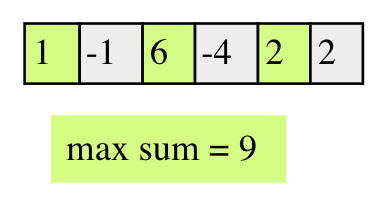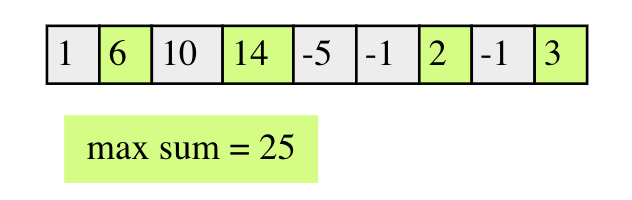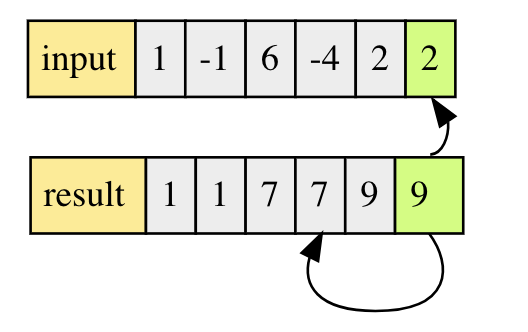# 向量

### 基本概念

1. 元素类型相同
• 元素顺序的存储在连续的存储空间中，每个元素有唯一的索引值
• 使用常数作为向量长度
2. 读写元素很方便，通过下标即可指定位置
• 只要确定了首地址，线性表中任意数据元素都可以随机访问

#### 顺序表类定义

class Array:public List<T>{
private:
T* list;
int maxSize; //向量实际的内存空间
int curLen; //向量当前长度，这个值小于maxSize，要预留一部分存储空间，放置频繁的内存开销
int position;
public:
Array(const int size){
maxSize = size;
list = new T[maxSize];
curLen = position = 0;
}
~MyList(){
delete []list;
list = nullptr;
}
void clear(){
delete []list;
list = nullptr;
position = 0;
list = new T[maxSize];
}
int length();
bool append(const T value);
bool insert(const int p, const T value);
bool remove(const int p);
bool setValue(const int p, const T value);
bool getValue(const int p, T& value);
bool getPos(int &p, const T value);
}


### 顺序表上的运算

• 插入运算
template<class T>
bool Array<T>::insert(const int p, const T value){
if(curLen >= maxSize){
//重新申请空间
//或者报错
return false;
}
if(p<0 || p>=curLen){
return false;
}
for(int i=curLen;i>p;i--){
list[i] = list[i-1];
}
list[p] = value;
curLen ++;

return true;
}


	x
|
a b c d e
a b x d e c


• 删除运算
template<class T>
bool Array<T>::delete(const int p){
if(curLen == 0){
return false;
}
if(p == 0 || p>=curLen){
return false;
}
for(int i=p; i<curLen-1; i++){
list[i] = list[i+1];
}
curLen--;

return true;
}


a b c | d e f g h


## 数组的应用

### 去重问题

1. 数组是否有序
2. 是否可以使用额外的辅助空间
3. 是否要求操作是inplace的，即去重操作需要在原数组内完成
4. 如果数组中有重复的元素有多个，是保留1个还是n个

class Solution {
public:
int removeDuplicates(vector<int>& nums) {
if(nums.size() <= 1 ){
return nums.size();
}
//two pointers
int write = 1;
}
}
return write;
}
};


int write = 2;
}
}


### 子数组最优解问题

1. 如果求连续子数组考虑使用滑动窗口
3. 动态规划

kadane算法的思路是遍历数组中的每个元素，以该元素结尾的最优subarray的和的计算公式为dp[i] = max(nums[i],dp[i-1]+nums[i])，意思是这个subarray要么是自己(例如前面都是负数，自己是正数)，要么是i-1位置的最大和subarray加上自己（比如前面都是正数，自己也是正数）。

class Solution {
public:
int maxSubArray(vector<int>& nums) {
vector<int> dp(nums.size(),0);
dp = nums;
int maxsum = dp;
for(int i=1;i<nums.size();i++){
dp[i] = max(nums[i],dp[i-1]+nums[i]);
maxsum = max(maxsum,dp[i]);
}
return maxsum;
}
};dp = arr;
dp = max(dp,arr);int find_max_sum_nonadjacent(vector<int>& a) {
if(a.size() == 0){
reutrn 0;
}
if(a.size() == 1){
return a;
}
//维护一个dp序列
vector<int> dp(a.size(),0);
dp = a;
dp = std::max(dp,a);
for(int i=2;i<a.size();i++){
dp[i] = max(dp[i-1],a[i]+dp[i-2]);
}
return dp.back();
}


### K-Sum问题

K Sum问题是数组中的经典问题了，其核心的问题为如何在一个数组中找到若干个数，使它们的和为k。根据这几个数之间的位置关系，又可以衍生出两个变种，一个是求连续的子数组和为k，另一个是求不连续的序列，使其和为k。

1. 对于非连续的元素可以考虑使用
• 先排序后双指针碰撞
• 使用hashmap做索引
• 将问题转化为k-1 sum， 比如3sum可转化为for循环+two sum
2. 如果要求数据是连续的，则可以考虑使用
• 双指针滑动窗口 + hashmap
• 暴利枚举

class Solution {
public:
int subarraySum(vector<int>& nums, int k) {
int count = 0;
for(int i=0;i<nums.size();i++){
int sum = 0;
for(int j=i;j<nums.size();j++){
sum += nums[j];
if(sum==k){
count += 1;
}
}
}
return count;
}
};


vector<int> arr = [1,-1,0,2];


vector<int> prefixSum = [1,0,0,2];


prefixSum[i] - prefixSum[x] = k; // x>=0 && x<i


class Solution {
public:
int subarraySum(vector<int>& nums, int k) {
vector<int> prefix;
int sum = 0;
for(int i=0;i<nums.size();i++){
sum+=nums[i];
prefix.push_back(sum);
}
int ans = 0;
unordered_map<int,int> um;
for(int i=0; i<nums.size();i++){
int prefixSum = prefix[i];
if(prefixSum == k){
ans+=1;
}
int target = prefixSum-k;
if(um.count(target)){
ans+=um[target];
}
um[prefixSum]++;
}
return ans;
}
};